#### The Form of the Finite Mixture Model

Suppose that you observe realizations of a random variable Y, the distribution of which depends on an unobservable random variable S that has a discrete distribution. S can occupy one of k states, the number of which might be unknown but is at least known to be finite. Since S is not observable, it is frequently referred to as a latent variable.

Let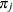denote the probability that S takes on state j. Conditional on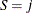, the distribution of the response Y is assumed to be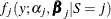. In other words, each distinct state j of the random variable S leads to a particular distributional form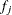and set of parameters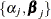for Y.

Let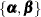denote the collection of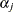and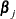parameters across all j = 1 to k. The marginal distribution of Y is obtained by summing the joint distribution of Y and S over the states in the support of S: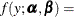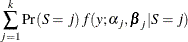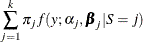This is a mixture of distributions, and theare called the mixture (or prior) probabilities. Because the number of states k of the latent variable S is finite, the entire model is termed a finite mixture (of distributions) model.

The finite mixture model can be expressed in a more general form by representing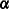andin terms of regressor variables and parameters with optional additional scale parameters for. The section Notation for the Finite Mixture Model develops this in detail.